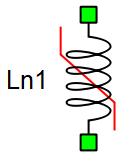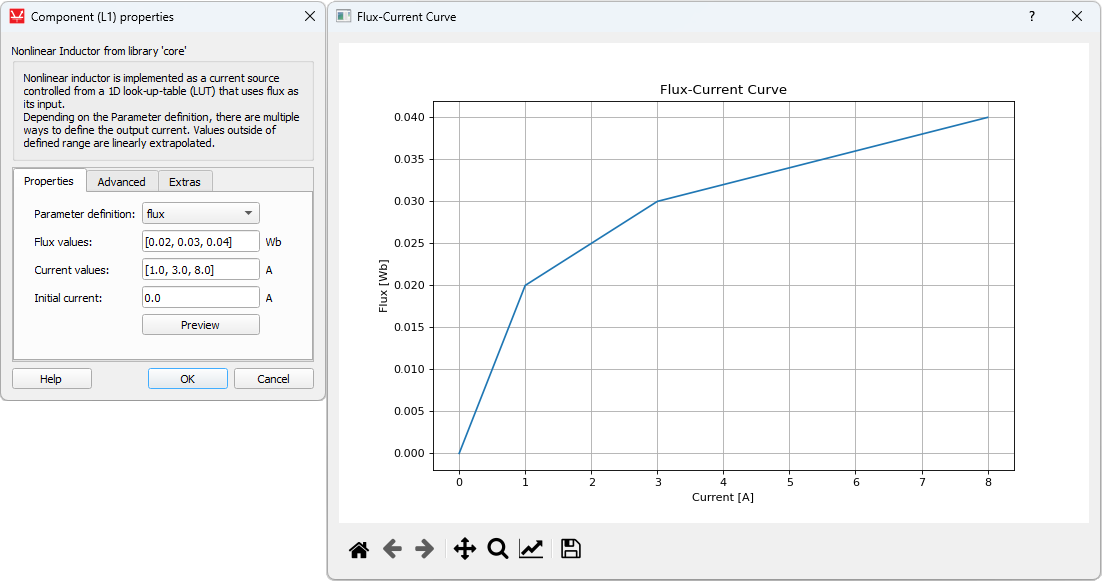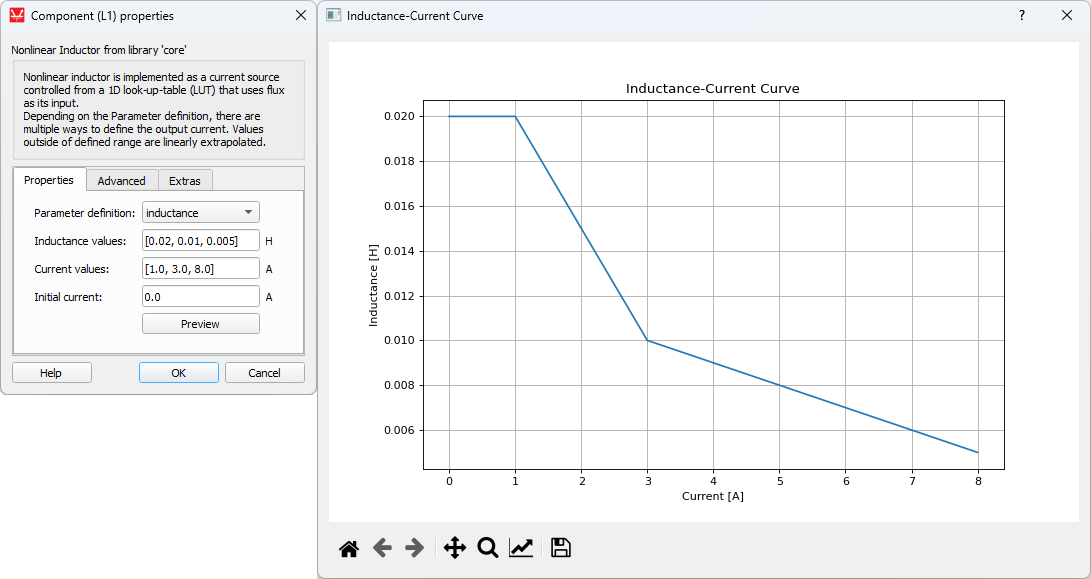# Nonlinear passive components

Description of nonlinear passive components in Schematic Editor

## Nonlinear inductor

Nonlinear inductor is available in Typhoon HIL's library of components. It is implemented as a controlled current source as a function of the inductor’s flux. Function i(Φ) (where i is the inductor current and Φ is the inductor flux) is defined through the properties window of the component. A look-up table (LUT) is generated based on the properties settings.

Table 1. Nonlinear passive components in HIL Toolbox
component component dialog window component parametersNonlinear inductor• Vector of flux values (Wb)
• Vector of inductance values (H)
• Vector of current values (A)
• Initial current (A)
• Curve preview button
• Enable snubber
• Snubber type
• Resistance
• Capacitance
• DCM optimization
The Parameter definition property provides a choice of three different parameter definition methods:
• The flux method uses a user-defined flux-current curve. Linear interpolation is applied to the flux values between the defined points, while linear extrapolation is applied in case a current value is outside of the specified range. The property window and the generated preview flux-current curve are shown in Figure 1.Figure 1. The property window and the flux-current curve
• The inductance method uses inductance and current vectors to calculate the fluxes, assuming that $\Phi \left(i\right)=Li$ . It is assumed that inductance has a constant value for currents smaller than the smallest current value in the current values vector. Linear interpolation is applied to the flux vector values that are calculated based on provided inductance and current values. The property window and the generated preview flux-current curve are shown in Figure 2.
• The inductance (dΦ/di) method uses inductance and current vectors to calculate the fluxes, assuming that $\Phi \left(I\right)={\int }_{0}^{I}Ldi$ . It is assumed that inductance has a constant value for currents smaller than the smallest current value in the current values vector. Linear interpolation is first applied to the inductance vector values in order to ensure a continuous function L(i). This function is integrated in order to get the flux-current curve for the LUT. The property window and the generated preview flux-current curve are shown in Figure 2.Figure 2. The property window and the inductance-current curve

The schematic diagram of the nonlinear inductor model is shown in Figure 3. In the electrical circuit, nonlinear inductor is represented as a current source. Voltage across the inductor is measured and integrated, the result of the integration being the inductor’s flux. Flux is used to address the LUT. The output of the look-up table is the inductor current which is fed to the controlled current source.